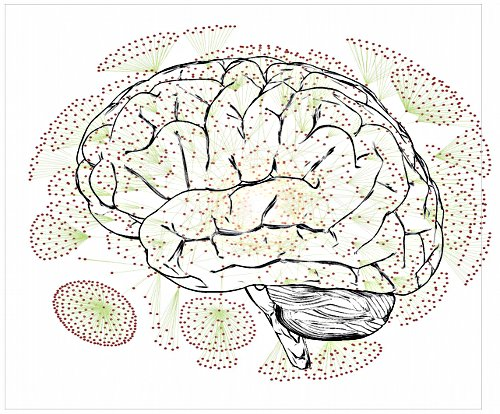# Neural Networks

[wpcol_1half id="" class="" style=""]

## Neural Networks

Computational neuroscience is a growing and recent field, compared to better theoretically defined and already explored fields such as mathematics or theoretical physics. A theorem can not be always demonstrated on a sheet of paper since analytical solutions are far from possible when speaking about modelling biology, which is everything except clearly defined and constrained. Approximations, hypotheses, numerical simulations and computational models are the sine qua none conditions for predictions and inferences in large-scale models of neuronal networks. Simulating those networks is crucial for understanding large-scale and generic dynamical properties that may emerge from the complex interactions between all neurons, and since analytical solutions are hardly tractable, one has to deal with numerical approaches. Section is divided as follow:

[/wpcol_1half]

[wpcol_1half_end id="" class="" style=""]Artistic view of a brain and schematic of its embeddde neural networks

[/wpcol_1half_end]